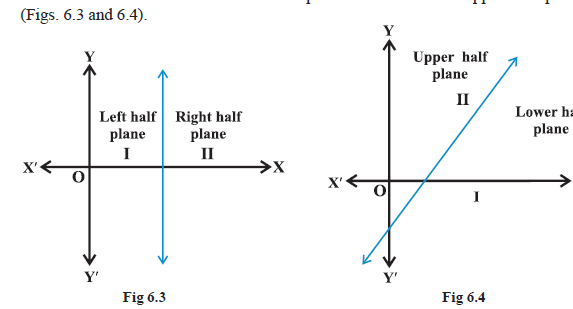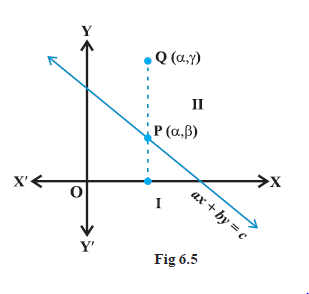Mathematics Graphical Solution of Linear Inequalities in Two Variables
Click for Only Video

### Topic Covered

star Cartesian plane into two parts
star Graphical Solution of Linear Inequalities in Two Variables

### Cartesian plane into two parts\color{purple} ✍️ We know that a line divides the Cartesian plane into two parts. Each part is known as a color(green)("half plane.")

\color{purple} ✍️ A color(green)("vertical line") will divide the plane in color(green)("left and right half planes") and a color(green)("non-vertical line") will divide the plane into color(green)("lower and upper half planes") (Figs. 6.3 and 6.4).

\color{purple} ✍️ A point in the Cartesian plane will either lie on a line or will lie in either of the half planes I or II.

\color{purple} ✍️ We shall now examine the relationship, if any, of the points in the plane and the inequalities ax + by < c or ax + by > c.

### Graphical Solution of Linear Inequalities in Two Variables\color{red} ✍️ Let us consider the line color(purple)(ax + by = c, \ \ a ≠ 0, b ≠ 0) .................................. (1)

color(red)("There are three possibilities namely :")

(i) color(blue)(ax + by = c) \ \ \ \ (ii) color(blue)(ax + by > c) \ \ \ \ \ (iii) color(blue)(ax + by < c.)

\color{red} ✍️ In case (i), clearly, all points (x, y) satisfying (i) lie on the line it represents and conversely.

\color{red} ✍️ Consider case (ii), color(red)("let us first assume that" \ \ b > 0.)

Consider a point color(blue)(P (α,β)) on the line ax + by = c, b > 0, so that aα + bβ = c. Take an arbitrary point Q (α , γ) in the half plane II (Fig 6.5).

● we interpret, γ > β

or b γ > bβ or aα + b γ > aα + bβ or aα + b γ > c i.e., Q(α, γ ) satisfies the inequality ax + by > c

● Thus, all the points lying in the half plane II above the line ax + by = c satisfies the inequality ax + by > c. Conversely, let (α, β) be a point on line ax + by = c and an arbitrary point Q(α, γ) satisfying

ax + by > c

so that aα + bγ > c

⇒ aα + b γ > aα + bβ

color(blue)(⇒ γ > β (as b > 0))

color(blue)("This means that the point (α, γ ) lies in the half plane II.")

\color{green} ★ \color{green} \mathbf(KEY \ POINTS)

"1" The region containing all the solutions of an inequality is called color(blue)("the solution region.")

2. In order to identify the half plane represented by an inequality, it is just sufficient to take any point (a, b) (not online) and check whether it satisfies the inequality or not.

If it satisfies, then the inequality represents the half plane and shade the region which contains the point, otherwise, the inequality represents that half plane which does not contain the point within it. For convenience, the point (0, 0) is preferred.

3. If an inequality is of the type color(blue)(ax + by ≥ c) or color(blue)(ax + by ≤ c,) then the points on the line color(blue)(ax + by = c) are also included in the solution region. So draw a dark line in the solution region.

4. If an inequality is of the form ax + by > c or ax + by < c, then the points on the line ax + by = c are not to be included in the solution region. So draw a broken or dotted line in the solution region.
Q 3131401322Solve 3x + 2y > 6 graphically.Solution:

Graph of 3x + 2y = 6 is given as dotted line in the Fig 6.8. This line divides the xy-plane in two half planes I and II. We select a point (not on the line), say (0, 0), which lies in one of the half planes (Fig 6.8) and determine if this point satisfies the given inequality, we note that 3 (0) + 2 (0) > 6 or 0 > 6 , which is false. Hence, half plane I is not the solution region of the given inequality. Clearly, any point on the line does not satisfy the given strict inequality. In other words, the shaded half plane II excluding the points on the line is the solution region of the inequality.
Q 3151401324Solve 3x - 6 ≥ 0 graphically in two dimensional plane.Solution:

Graph of 3x – 6 = 0 is given in the
We select a point, say (0, 0) and substituting it in given inequality, we see that: 3 (0) – 6 ≥ 0 or – 6 ≥ 0 which is false.
Thus, the solution region is the shaded region on the right hand side of the line x = 2.
Q 3171401326Solve y < 2 graphically.Solution:

Graph of y = 2 is given in the Fig 6.10. Let us select a point, (0, 0) in lower half plane I and putting y = 0 in the given inequality, we see that 1 × 0 < 2 or 0 < 2 which is true. Thus, the solution region is the shaded region below the line y = 2. Hence, every point below the line (excluding all the points on the line) determines the solution of the given inequality.